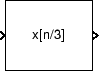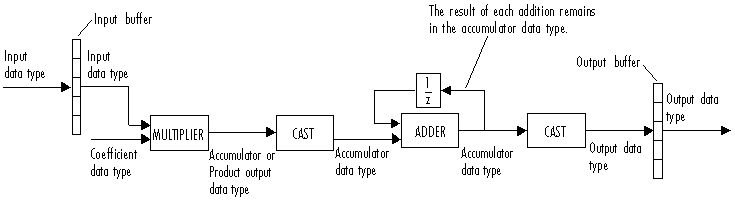Documentation

### This is machine translation

Mouseover text to see original. Click the button below to return to the English version of the page.

# FIR Interpolation

Upsample and filter input signals

## Library

Filtering / Multirate Filters

`dspmlti4`## Description

The FIR Interpolation block resamples the discrete-time input at a rate L times faster than the input sample rate, where L is the integer value you specify for the Interpolation factor parameter. To do so, the block implements a polyphase filter structure and performs the following operations:

• Upsamples each channel of the input to a higher rate by inserting L–1 zeros between samples.

• Filters each channel of the upsampled data using a direct-form FIR filter.

The block uses a polyphase filter implementation because it is more efficient than straightforward upsample-then-filter algorithms. See Fliege  for more information.

You can use the FIR Interpolation block inside triggered subsystems when you set the Rate options parameter to ```Enforce single-rate processing```.

### Specifying the Filter Coefficients

To specify the filter coefficients, select the mode you want the FIR Interpolation block to operate in. Select the mode in the Coefficient source group box.

• Dialog parameters — Enter information about the filter, such as coefficients in the block dialog box.

• Input port — Specify the filter coefficients as an input to the block. Coefficient values are tunable (can change during simulation), while their properties must remain constant.

• Filter object — Specify the filter using a `dsp.FIRInterpolator` System object™.

• Auto (default) — Choose the filter coefficients of an FIR Nyquist filter, predesigned for the interpolation factor specified in the block dialog box.

When you select Dialog parameters, you use the FIR filter coefficients parameter to specify the numerator coefficients of the FIR filter transfer function H(z).

`$H\left(z\right)=B\left(z\right)={b}_{1}+{b}_{2}{z}^{-1}+\dots +{b}_{m}{z}^{-\left(m-1\right)}$`

You can generate the FIR filter coefficient vector, ```[b(1) b(2) ... b(m)]```, using one of the DSP System Toolbox™ filter design functions such as `designMultirateFIR`, `firnyquist`, `firhalfband`, `firgr` or `firceqrip`.

The filter you specify must be a lowpass filter with a length greater than the interpolation factor (m>L) and a normalized cutoff frequency no greater than `1`/L. The block internally initializes all filter states to zero.

When you select Auto, the block designs an FIR interpolator with the interpolation factor specified in Interpolation factor. The `designMultirateFIR` function designs the filter and returns the coefficients used by the block. For more information on the filter design, see Orfanidis .

### Frame-Based Processing

When you set the Input processing parameter to `Columns as channels (frame based)`, the block resamples each column of the input over time. In this mode, the block can perform either single-rate or multirate processing. You can use the Rate options parameter to specify how the block resamples the input:

• When you set the Rate options parameter to `Enforce single-rate processing`, the input and output of the block have the same sample rate. To interpolate the output while maintaining the input sample rate, the block resamples the data in each column of the input such that the frame size of the output (Mo) is L times larger than that of the input (Mo = Mi*L).

For an example of single-rate FIR Interpolation, see Example 1 — Single-Rate Processing.

• When you set the Rate options parameter to `Allow multirate processing`, the input and output of the FIR Interpolation block are the same size. However, the sample rate of the output is L times faster than that of the input. In this mode, the block treats an Mi-by-N matrix input as N independent channels. The block interpolates each column of the input over time by keeping the frame size constant (Mi=Mo), while making the output frame period (Tfo) L times shorter than the input frame period (Tfo = Tfi/L).

See Example 2 — Multirate Frame-Based Processing for an example that uses the FIR Interpolation block in this mode.

### Sample-Based Processing

When you set the Input processing parameter to `Elements as channels (sample based)`, the block treats an M-by-N matrix input as M*N independent channels, and interpolates each channel over time. The output sample period (Tso) is L times shorter than the input sample period (Tso = Tsi/L), while the input and output sizes remain identical.

### Latency

When you run your models in Simulink® `SingleTasking` mode or set the Input processing parameter to ```Columns as channels (frame based)``` and the Rate options parameter to `Enforce single-rate processing`, the FIR Interpolation block always has zero-tasking latency. Zero-tasking latency means that the block propagates the first filtered input sample (received at time t=`0`) as the first output sample. That first output sample is then followed by L`1` interpolated values, the second filtered input sample, and so on.

The only time the FIR Interpolation block exhibits latency is when you set the Rate options parameter set to ```Allow multirate processing``` and run your models in Simulink `MultiTasking` mode. The amount of latency for multirate, multitasking operation depends on the setting of the Input processing parameter, as shown in the following table.

Input processingLatency

```Elements as channels (sample based)```

L samples

```Columns as channels (frame based)```

L frames (Mi samples per frame)

When the block exhibits latency, the default initial condition is zero. Alternatively, you can use the Output buffer initial conditions parameter to specify a matrix of initial conditions containing one value for each channel or a scalar initial condition to be applied to all channels. The block scales the Output buffer initial conditions by the Interpolation factor and outputs the scaled initial conditions until the first filtered input sample becomes available.

When the block is in sample-based processing mode, the block outputs the scaled initial conditions at the start of each channel, followed immediately by the first filtered input sample, then L–1 interpolated values, and so on.

When the block is in frame-based processing mode and using the default initial condition of zero, the first Mi*L output rows contain zeros, where Mi is the input frame size. The first filtered input sample (first filtered row of the input matrix) appears in the output as sample Mi*L+`1`. That value is then followed by L–1 interpolated values, the second filtered input sample, and so on.

### Fixed-Point Data Types

The following diagram shows the data types used within the FIR Interpolation block for fixed-point signals.You can set the coefficient, product output, accumulator, and output data types in the block dialog box as discussed in Dialog Box section. This diagram shows that input data is stored in the input buffer with the same data type and scaling as the input. The block stores filtered data and any initial conditions in the output buffer using the output data type and scaling that you set in the block dialog box.

When at least one of the inputs to the multiplier is real, the output of the multiplier is in the product output data type. When both inputs to the multiplier are complex, the result of the multiplication is in the accumulator data type. For details on the complex multiplication performed by this block, see Multiplication Data Types.

### Note

When the block input is fixed point, all internal data types are signed fixed point.

## Examples

### Example 1 — Single-Rate Processing

In the ex_firinterpolation_ref2, the FIR Interpolation block interpolates a single-channel input with a frame size of `16`. Because the block is doing single-rate processing and the Interpolation factor parameter is set to `4`, the output of the FIR Interpolation block has a frame size of `64`. As shown in the following figure, the input, and output of the FIR Interpolation block have the same sample rate.### Example 2 — Multirate Frame-Based Processing

In the ex_firinterpolation_ref1, the FIR Interpolation block interpolates a single-channel input with a frame period of 1 second (Sample time = `1/64` and Samples per frame = `64`). Because the block is doing multirate frame-based processing and the Interpolation factor parameter is set to `4`, the output of the FIR Interpolation block has a frame period of `0.25` seconds. As shown in the following figure, the input and output of the FIR Interpolation block have the same frame size, but the sample rate of the output is `1/4` times that of the input.### Example 3

The `ex_polyphaseinterp` model illustrates the underlying polyphase implementations of the FIR Interpolation block. Run the model, and view the results on the scope. The output of the FIR Interpolation block matches the output of the Polyphase Interpolation Filter block.

### Example 4

The `ex_mrf_nlp` model illustrates the use of the FIR Interpolation block in a number of multistage multirate filters.

## Dialog Box

### Coefficient Source

The FIR Interpolation block can operate in four different modes. Select the mode in the Coefficient source group box.

• Dialog parameters — Enter information about the filter, such as coefficients, in the block mask.

• Input port — Specify the filter coefficients with a Num input port. The Num input port appears when you select the Input port option. Coefficient values obtained through Num are tunable (can change during simulation), while their properties must remain constant.

• Filter object — Specify the filter using a `dsp.FIRInterpolator` System object.

• Auto (default) — Choose the coefficients of an FIR Nyquist filter, predesigned for the Interpolation factor specified in the block dialog box.

Different items appear on the FIR Interpolation block dialog box depending on whether you select Dialog parameters, Input port, Filter object, or Auto in the Coefficient source group box.

## References

 Fliege, N. J. Multirate Digital Signal Processing: Multirate Systems, Filter Banks, Wavelets. West Sussex, England: John Wiley & Sons, 1994.

 Orfanidis, Sophocles J. Introduction to Signal Processing. Upper Saddle River, NJ: Prentice-Hall, 1996.

## Supported Data Types

PortSupported Data Types

Input

• Double-precision floating point

• Single-precision floating point

• Fixed point

• 8-, 16-, and 32-bit signed integers

• 8-, 16-, and 32-bit unsigned integers

Output

• Double-precision floating point

• Single-precision floating point

• Fixed point

• 8-, 16-, and 32-bit signed integers

• 8-, 16-, and 32-bit unsigned integers

 FIR Decimation DSP System Toolbox FIR Rate Conversion DSP System Toolbox FIR Halfband Interpolator DSP System Toolbox FIR Halfband Decimator DSP System Toolbox IIR Halfband Interpolator DSP System Toolbox IIR Halfband Decimator DSP System Toolbox CIC Compensation Interpolator DSP System Toolbox CIC Compensation Decimator DSP System Toolbox Upsample DSP System Toolbox CIC Interpolation DSP System Toolbox Digital Down-Converter DSP System Toolbox Digital Up-Converter DSP System Toolbox `dsp.FIRInterpolator` DSP System Toolbox `dsp.CICCompensationInterpolator` DSP System Toolbox `dsp.FIRHalfbandInterpolator` DSP System Toolbox `firnyquist` DSP System Toolbox `firhalfband` DSP System Toolbox `firgr` DSP System Toolbox `firceqrip` DSP System Toolbox

Watch now